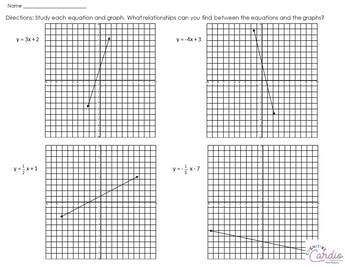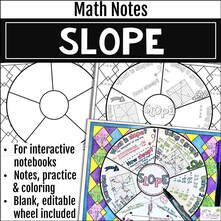# Teaching Slope

## Discovering Slope in 6th grade Math

I’ve never had the opportunity to teach in 6th grade slope before this year.

This year is a first for several topics, among which was graphing functions – we did this last week, by using function tables to generate points, and I mentioned positive and negative slopes in passing at that time.

In getting more specific about slope, I knew I didn’t want to just tell the students about slope (and about y-intercept) – I wanted them to figure out how the equation of a line can help them understand aspects of the graph of the line. But, I didn’t know quite what to do.

## Slope ActivityAfter some thought, here’s what I decided to do:
​I created a simple worksheet with four equations and their graphs.
1) I simply asked students to find relationships between the numbers (and symbols) in the equations and the graphs of the equations.

• I didn’t give much more direction than that.
• I had them each think about this, study the equations and graphs, and write their observation on their papers, without discussing with anyone, for about 5 minutes.

2) Then, I had them choose a partner to discuss their observations with, and to search for more ideas,

• This step was also about five minutes.
• As students discussed, I circulated, listened, and asked questions.
• For the most part, students had written down how the negative/positive sign in front of the x relates to the slope.
• Many had identified the “added or subtracted number” as the y-intercept.
• Some had noticed that when the coefficient is higher, the slope of the line is steeper.

3) Next, I re-paired the students using popsicle sticks, to allow them to share more ideas. At this point, I wrote on the board:

• “# that is added or subtracted”
• “# in front of the x,”

I asked students to try to figure out what these numbers could tell them about the line (if they hadn’t figured it out already).

• There weren’t many students who made the connection that the slope tells how far to move horizontally and vertically between points, but there were several student whose observation was that the “m” is “how far apart” the points on the line were (they identified the points as where the line crossed the intersection of grid lines – I didn’t put points on the lines for them).
• After the second pairing, I asked student to write their observations on the board and then we went through and discussed whether they were correct or not.

4) We looked at the same lines graphed on the Smartboard, and we went through what the “m” tells us – we started with the fractional slopes and moved to the whole number slopes.

## Slope Activity Evaluation

In all, the entire lesson took about 35 minutes. I was really happy with the students’ perseverance (for the most part) in trying to find what I wanted them to find:)

I really enjoyed their “a-ha” moments!

One “mistake” I made in the equations was that both equations with negative slopes also had negative y-intercepts…this led some students to incorrect conclusions, so I changed that for next year. The fixed worksheet can be downloaded by selecting the button below, if you’d like to use it:)

Today’s thinking about slope is my favorite kind of day:-)

How do you introduce slope to your 6th grade or middle school math students?

## Resource for Teaching and Practicing Slope:Since originally writing this post, I’ve created a Slope Notes Wheel to help teach students about slope. They love it:-) This wheel is great for students to keep in their notebooks as a reference!

## EllieWelcome to Cognitive Cardio Math! I’m Ellie, a wife, mom, grandma, and dog ‘mom,’ and I’ve spent just about my whole life in school! With nearly 30 years in education, I’ve taught:

• All subject areas in 4th and 5th grades
• Math, ELA, and science in 6th grade (middle school)

I’ve been creating resources for teachers since 2012 and have worked in the elearning industry for about five years as well!